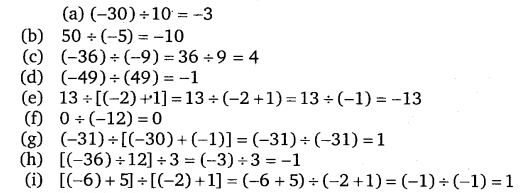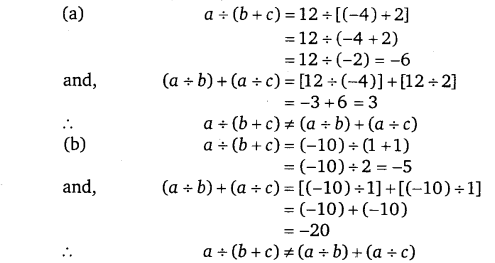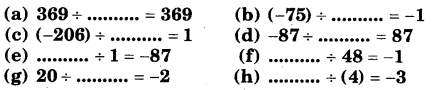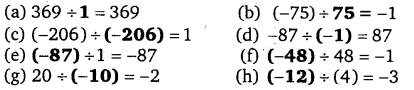# Class 7 Maths NCERT Solutions for Chapter 1 Integers Chapter 1 Integers EX – 1.4

## Integers

Question 1.
Evaluate each of the following:Solution.Question 2.
values of a, b and c.
(a) a = 12, b = -4, c = 2
(b) a = (-10), b = 1, c = 1

Solution.Question 3.
Fill in the blanks :Solution.Question 4.
Write five pairs of integers (a, b) such that$a\div b$ = -3. One such pair is (6, – 2) because$6\div \left( -2 \right) =\left( -3 \right)$ .

Solution.
Five pairs of integers (a, b) such that a + b = -3 are : (-6,2), (-9, 3), (12,-4), (21,-7), (-24, 8)
Note : We may write many such pairs of integers.

Question 5.
The temperature at 12 noon was 10°C above zero. If it decreases at the rate of 2 °C per hour until mid-night, at what time would the temperature be 8 °C below zero? What would be the temperature at mid-night?

Solution.
Difference in temperatures +10 °C and -8
= [10 – (-8)] °C = (10 + 8)° C = 18 °C
Decrease in temperature in one hour = 2°C
Number of hours taken to have temperature 8 °C below zero$=\frac { Total\quad decrease }{ Decrease\quad in\quad one\quad hour }$$=\frac { 18 }{ 2 }$
So, at 9 P.M., the temperature will be 8 °C below zero
Temperature at mid-night = 10 °C – (2 x 12) °C
= 10°C – 24 °C = -14 °C

Question 6.
In a class test (+ 3) marks are given for every correct answer and (- 2) marks are given for every incorrect answer and no marks for not attempting any question, (i) Radhika scored 20 marks. If she has got 12 correct answers, how many questions has she attempted incorrectly? (ii) Mohini scores – 5 marks in this test though she has got 7 correct, answers. How many questions has she attempted incorrectly?

Solution.
(i) Marks given for 12 correct answers at the rate of + 3 marks for each answer = 3 x 12 = 36 Radhika’s score = 20 marks
∴ Marks deducted her for incorrect answers = 20 – 36 = -16
Marks given for one incorrect answer = -2
Number of incorrect answers$=\left( -16 \right) \div \left( -2 \right) =8$
(ii) Marks given for 7 correct answers at the rate of + 3 marks for each answer = 3 x 7 = 21 Mohini’s score = -5
∴ Marks deducted for incorrect answers
= – 5 – 21 = -26
Marks given for one incorrect answer = -2
∴ Number of incorrect answers$=\left( -26 \right) \div \left( -2 \right) =13$

Question 7.
An elevator descends into a mine shaft at the rate of 6 m/min. If the descent starts from 10 m above the ground level, how long will it take to reach – 350 m.

Difference in heights at two positions = 10 m – (- 350 m) = 360 m
Rate of descent = 6 m/minute
∴ Time taken$=\left( 360 \right) \div \left( 6 \right)$ minutes
= 60 minutes = 1 hour
Hence, the elevator will take 1 hour to reach – 350 m.